# 软件姿态解算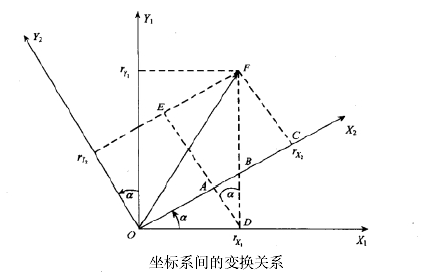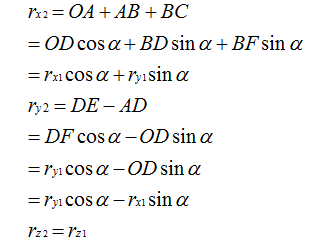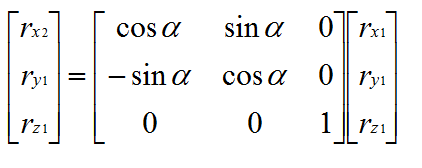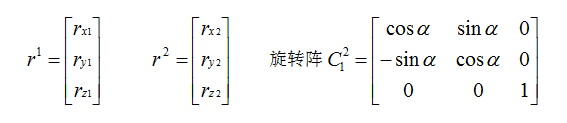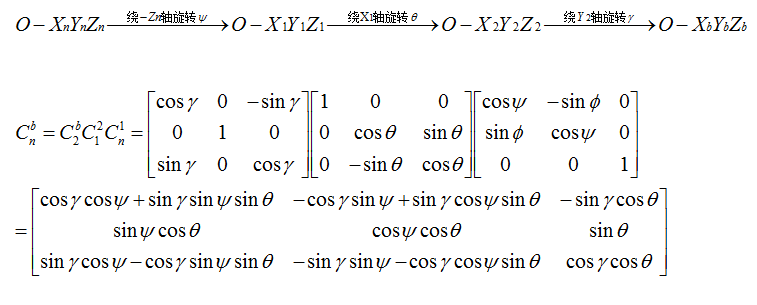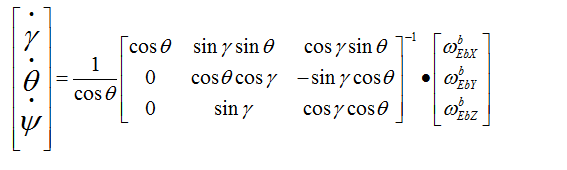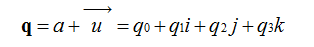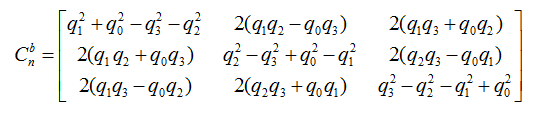void IMUupdate(float gx, float gy, float gz, float ax, float ay, float az) {
float norm;
float vx, vy, vz;
float ex, ey, ez;

norm = sqrt(ax*ax + ay*ay + az*az);
ax = ax / norm;
ay = ay / norm;
az = az / norm;


// estimated direction of gravity
vx = 2*(q1*q3 - q0*q2);
vy = 2*(q0*q1 + q2*q3);
vz = q0*q0 - q1*q1 - q2*q2 + q3*q3;


axyz是机体坐标参照系上，加速度计测出来的重力向量，也就是实际测出来的重力向量。

axyz是测量得到的重力向量，vxyz是陀螺积分后的姿态来推算出的重力向量，它们都是机体坐标参照系上的重力向量。

// integral error scaled integral gain
exInt = exInt + ex*Ki;
eyInt = eyInt + ey*Ki;
ezInt = ezInt + ez*Ki;


// integral error scaled integral gain
exInt = exInt + ex*Ki;
eyInt = eyInt + ey*Ki;
ezInt = ezInt + ez*Ki;

gx = gx + Kp*ex + exInt;
gy = gy + Kp*ey + eyInt;
gz = gz + Kp*ez + ezInt;// integrate quaternion rate and normalise
q0 = q0 + (-q1*gx - q2*gy - q3*gz)*halfT;
q1 = q1 + (q0*gx + q2*gz - q3*gy)*halfT;
q2 = q2 + (q0*gy - q1*gz + q3*gx)*halfT;
q3 = q3 + (q0*gz + q1*gy - q2*gx)*halfT;Q_ANGLE.Yaw = atan2(2 * q1 * q2 + 2 * q0 * q3, -2 * q2*q2 - 2 * q3* q3 + 1)* 57.3; // yaw
Q_ANGLE.Y  = asin(-2 * q1 * q3 + 2 * q0* q2)* 57.3; // pitch
Q_ANGLE.X = atan2(2 * q2 * q3 + 2 * q0 * q1, -2 * q1 * q1 - 2 * q2* q2 + 1)* 57.3; // roll